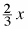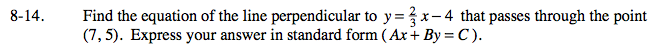### Home > PC > Chapter 8 > Lesson 8.1.1 > Problem8-14

8-14.

Find the equation of the line perpendicular to y =− 4 that passes through the point (7, 5). Express your answer in standard form (Ax + By = C). Homework Help ✎The ⊥ slope is negative the reciprocal of the line slope.

Use the point - slope form first to find the equation of the ⊥ line. Then put it in standard form.